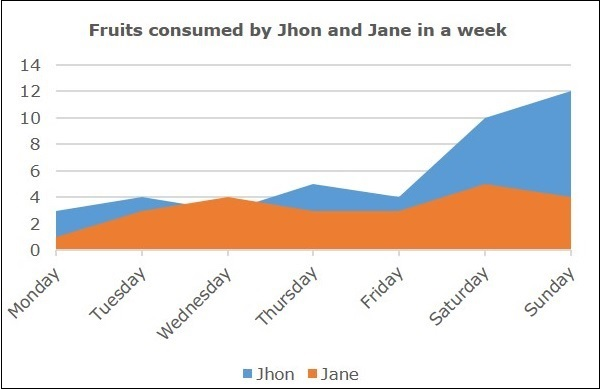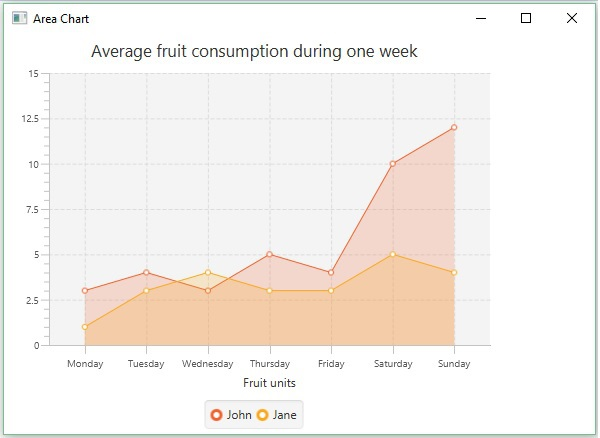# JavaFX - Area Chart

Area charts are used to draw area based charts. It plots the area between the given series of points and the axis. In general, this chart is used to compare two quantities.

Following is an Area chart depicting the number of fruits consumed by two people in a week.In JavaFX, an Area chart is represented by a class named AreaChart. This class belongs to the package javafx.scene.chart. By instantiating this class, you can create an AreaChart node in JavaFX.

## Steps to Generate Area Chart

To generate an area chart in JavaFX, follow the steps given below.

### Step 1: Creating a Class

Create a Java class and inherit the Application class of the package javafx.application and implement the start() method of this class as follows.

```public class ClassName extends Application {
@Override
public void start(Stage primaryStage) throws Exception {
}
}
```

### Step 2: Defining the Axis

Define the X and Y axis of the area chart and set labels to them. In our example, X axis represents the days in a week and the y axis represents the units of fruits consumed.

```//Defining the X axis
CategoryAxis xAxis = new CategoryAxis();

//Defining the y Axis
NumberAxis yAxis = new NumberAxis(0, 15, 2.5);
yAxis.setLabel("Fruit units");
```

### Step 3: Creating the Area Chart

Create a line chart by instantiating the class named AreaChart of the package javafx.scene.chart. To the constructor of this class, pass the objects representing the X and Y axis created in the previous step.

```//Creating the Area chart
AreaChart<String, Number> areaChart = new AreaChart(xAxis, yAxis);
areaChart.setTitle("Average fruit consumption during one week");
```

### Step 4: Preparing the data

Instantiate the XYChart.Series class. Then add the data (a series of, x and y coordinates) to the Observable list of this class as follows −

```//Prepare XYChart.Series objects by setting data
XYChart.Series series1 = new XYChart.Series();
series1.setName("John");

XYChart.Series series2 = new XYChart.Series();
series2.setName("Jane");

```

### Step 5: Add Data to the Area Chart

Add the data series prepared in the previous step to the area chart as follows −

```//Setting the XYChart.Series objects to area chart
```

### Step 6: Creating a Group Object

In the start() method, create a group object by instantiating the class named Group, which belongs to the package javafx.scene.

Pass the AreaChart (node) object, created in the previous step as a parameter to the constructor of the Group class. This should be done in order to add it to the group as follows −

```Group root = new Group(areaChart);
```

### Step 7: Creating a Scene Object

Create a Scene by instantiating the class named Scene, which belongs to the package javafx.scene. To this class, pass the Group object (root) created in the previous step.

In addition to the root object, you can also pass two double parameters representing height and width of the screen, along with the object of the Group class as follows.

```Scene scene = new Scene(group ,600, 300);
```

### Step 8: Setting the Title of the Stage

You can set the title to the stage using the setTitle() method of the Stage class. The primaryStage is a Stage object, which is passed to the start method of the scene class as a parameter.

Using the primaryStage object, set the title of the scene as Sample Application as follows.

```primaryStage.setTitle("Sample Application");
```

### Step 9: Adding Scene to the Stage

You can add a Scene object to the stage using the method setScene() of the class named Stage. Add the Scene object prepared in the previous steps using the following method.

```primaryStage.setScene(scene);
```

### Step 10: Displaying the Contents of the Stage

Display the contents of the scene using the method named show() of the Stage class as follows.

```primaryStage.show();
```

### Step 11: Launching the Application

Launch the JavaFX application by calling the static method launch() of the Application class from the main method as follows.

```public static void main(String args[]){
launch(args);
}
```

### Example

The following table depicts the number of fruits consumed by John and Jane in a week.

Day of the Week Fruits consumed by John Fruits consumed by Jane
Monday 3 1
Tuesday 4 3
Wednesday 3 4
Thursday 5 3
Friday 4 3
Saturday 10 5
Sunday 12 4

Following is a Java program which generates an area chart, depicting the above data using JavaFX.

Save this code in a file with the name AreaChartExample.java.

```import javafx.application.Application;
import javafx.scene.Group;
import javafx.scene.Scene;
import javafx.scene.chart.AreaChart;
import javafx.scene.chart.CategoryAxis;
import javafx.stage.Stage;
import javafx.scene.chart.NumberAxis;
import javafx.scene.chart.XYChart;

public class AreaChartExample extends Application {
@Override
public void start(Stage stage) {
//Defining the X axis
CategoryAxis xAxis = new CategoryAxis();

//defining the y Axis
NumberAxis yAxis = new NumberAxis(0, 15, 2.5);
yAxis.setLabel("Fruit units");

//Creating the Area chart
AreaChart<String, Number> areaChart = new AreaChart(xAxis, yAxis);
areaChart.setTitle("Average fruit consumption during one week");

//Prepare XYChart.Series objects by setting data
XYChart.Series series1 = new XYChart.Series();
series1.setName("John");

XYChart.Series series2 = new XYChart.Series();
series2.setName("Jane");

//Setting the XYChart.Series objects to area chart

//Creating a Group object
Group root = new Group(areaChart);

//Creating a scene object
Scene scene = new Scene(root, 600, 400);

//Setting title to the Stage
stage.setTitle("Area Chart");

stage.setScene(scene);

//Displaying the contents of the stage
stage.show();
}
public static void main(String args[]){
launch(args)
}
}
```

Compile and execute the saved java file from the command prompt using the following commands.

```javac AreaChartExample.java
java AreaChartExample
```

On executing, the above program generates a JavaFX window displaying an area chart as shown below.javafx_charts.htm